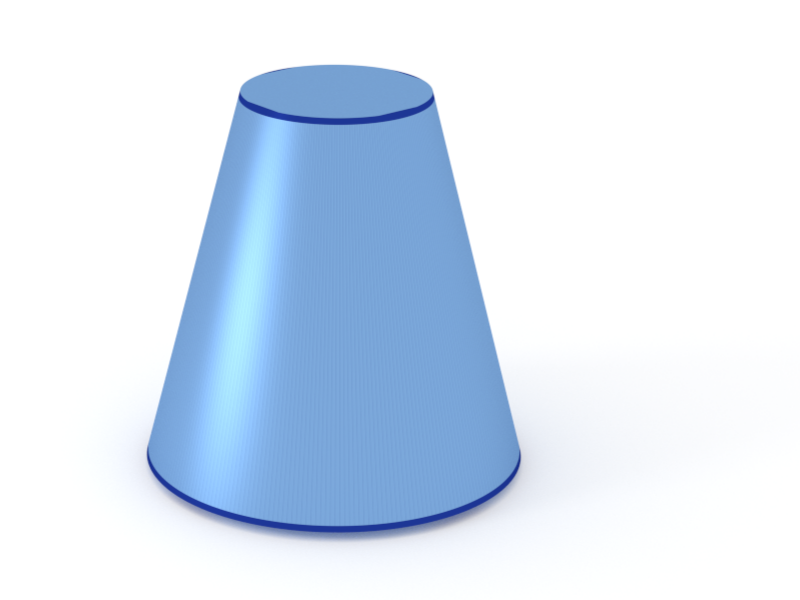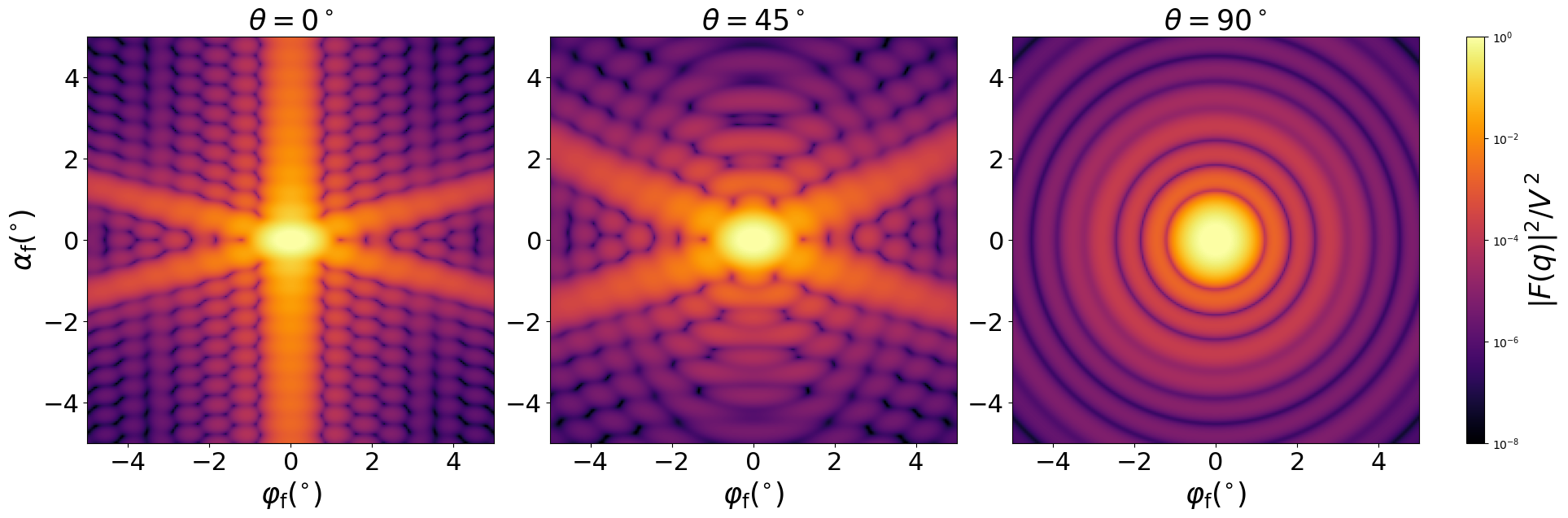### Cone

A truncated cone (frustum) with circular base.#### Constructor

Cone(R, H, alpha)


Parameters:

• H, height
• alpha, angle $\alpha$ between the base and a side.

Constraint:

$H \le R \tan\alpha$

#### Usage

As for any other Form factor.

#### Implementation

Class Cone inherits from the interface class IFormFactor .

Computation involves numerical integration in radial direction, $$F(\mathbf{q})=2\pi \exp(i \tilde{q_{z}} R) \tan\alpha \int_{R_H}^R d\rho\thinspace \rho^2 \frac{J_1(q_{\parallel}\rho)}{q_{\parallel}\rho} e^{-i\tilde{q}_z \rho},$$

with notation $$\quad R_{H} := R-\dfrac{H}{\tan\alpha}, \quad q_{||} := \sqrt{q_{x}^2 + q_{y}^2}, \quad \tilde{q_{z}} := q_{z} \tan\alpha.$$

Volume has been validated against $$V=\dfrac{\pi}{3}\tan\alpha \left( R^3 - R_H^3\right).$$

More special:

#### Example

Scattering by uncorrelated, oriented cones for horizontal incidence. Rotation around $y$ axis:Generated by Examples/ff/Cone.py .

#### History

Agrees with “Cone” form factor in IsGISAXS [manual, Eq. 2.27; Renaud 2009, Eq. 225], except for a substitution $z\to\rho$ in our expression for $F$.